Scientific Papers

# Dissimilarity-based hypothesis testing for community detection in heterogeneous networks## 1 Introduction

The theory of complex networks has emerged as a powerful tool for studying complex systems. Networks represent interactions between units within a system, with vertices denoting systematic units and edges capturing their interactions . With the increasing availability of real-world data, researchers have been able to conduct studies across various fields. One crucial aspect in these studies is the identification of community structure, where individuals or entities are organized into distinct groups. This task, commonly referred to as community detection , shares similarities with graph clustering. Although numerous algorithms have been proposed for community detection including clustering algorithms [3, 4], modularity-based algorithms [5, 6], and dynamic algorithms [7, 8], no single algorithm performs well across all types of networks [9, 10]. Consequently, there is a persistent demand for a general and efficient method for community detection.

From a probabilistic perspective, vertices belonging to the same community are more likely to be connected compared to those in different communities. Therefore, the stochastic block model (SBM)  has been widely employed for community detection. The SBM offers a theoretical framework for studying detection thresholds and developing corresponding algorithms. A notable contribution by Decelle et al.  introduced the concept of a phase transition for community detection at the Kesten–Stigum threshold, leading to various investigations into different transition thresholds under varying recovery conditions [13, 14]. Furthermore, numerous algorithms have been proposed for the SBM, often tailored to specific research questions or the characteristics of the system under study. These algorithms encompass spectral methods [15, 16], semi-definite programming methods , profile-likelihood maximization , and pseudo-likelihood maximization . Of particular interest, Peixoto [20, 21] approached the SBM from a microcanonical perspective, focusing on the number of edges rather than their connection probabilities. This alternative viewpoint offered valuable insights into the SBM.

The standard SBM assumes that vertices within the same community are stochastically equivalent and possess the same expected degree, which does not align with real-world networks where the presence of prominent “hubs” is widespread. To tackle this limitation, Karrer and Newman  introduced the degree-corrected SBM (DCSBM) by incorporating vertex-specific “degree parameters” that multiply the edge probability between vertices i and j. Building upon this concept, numerous studies have focused on utilizing the DCSBM for community detection. Zhao et al.  established a comprehensive theory for assessing the consistency of community detection in the context of the DCSBM. They also compared various community detection criteria applicable to both the SBM and DCSBM. Chen et al.  proposed a method based on convex programming relaxation of modularity maximization and developed a weighted 1-norm k-medoids algorithm within the DCSBM framework. In contrast, Gao et al.  derived the misclassification proportion by evaluating asymptotic minimax risks, which depend on the degree parameter, community size, and connection parameter. It is important to note that all of these algorithms presuppose prior knowledge regarding the number of communities.

In practical scenarios, the only information available to us is the set of vertices and the set of edges, indicating which vertices are connected to each other and which are not. Consequently, determining the appropriate number of communities becomes a challenging task. To the best of our knowledge, existing approaches have primarily focused on the SBM framework. One direction involves initially detecting the optimal community structure for different numbers of communities and then using methods such as minimum description length , the Akaike information criterion , or the Bayesian information criterion  to penalize the model parameters. Another direction involves developing hypothesis tests to determine the number of communities, considering aspects such as asymptotic consistency  or the principal eigenvalue of a normalized adjacency matrix . However, both of these approaches suffer from certain limitations. They either require considerable time for large networks or may underestimate or overestimate the number of communities.

The goal of this paper is to simultaneously uncover the number of communities and the corresponding structure in heterogeneous networks in an efficient way. To this end, we propose a novel hypothesis test based on graph dissimilarity, which incorporates three distribution functions of the vertex distance, clustering coefficient, and alpha-centrality. The null hypothesis is assuming that the original network is a one-block DCSBM, i.e., the degree-corrected Erdös–Rényi graph (DCERG), from which one can estimate the connecting parameter and the degree parameter. Then, we compute the dissimilarity between the original network and the posterior DCERG and use the kernel density estimation (KDE) to formulate the dissimilarity distribution among DCERGs generated by the same parameters. If the hypothesis is rejected, we split the network by the bipartitioning algorithm until each subgraph accepts the hypothesis.

## 2 Hypothesis test

The standard SBM finds its origins in the realms of machine learning and statistics literature. Within theoretical computer science, it is commonly referred to as a planted partition model , and in mathematical contexts, it is acknowledged as an inhomogeneous random graph model . This probabilistic generative model for random graphs with community structures seamlessly blends the rigidity of a block model with a stochastic component. It stands as a benchmark in the challenging task of recovering community structures from network data.

To introduce the SBM, we begin with an unweighted and undirected graph denoted as G, consisting of N-labeled vertices that are organized into K blocks. The connections among these K nodes are represented by the adjacency matrix A, where aij = 1 if there exists an edge between nodes i and j, and 0 otherwise. It is important to note that self-connections are not allowed, aii = 0. For each node i, we assign a label bi to represent its membership in a particular community. Consequently, each vertex i ∈ [N] belongs to a block determined by the prior probability pj with j ∈ [K], and these probabilities satisfy the normalization $∑j=1Kpj=1$. Additionally, we introduce W as a K × K matrix, where each element wst represents the probability of connectivity between one vertex in block s and the other in block t. With these definitions, we can now express the conditional expectation of the adjacency matrix A given the block assignments b as follows:

When all labels are identical, the model simplifies to the classic Erdös–Rényi graph (ERG) , where meaningful reconstruction of communities becomes unfeasible. In the context of real-world networks, the model can be adjusted by maximizing this expectation concerning vertex labels b. The primary objective of the community detection problem is to accurately reconstruct these labels.

In the standard SBM, the connecting probabilities between any two vertices within the same block are uniform. In such a configuration, the emergence of “hubs” becomes unlikely, and maximizing the log-likelihood function based on it tends to partition the graph into two groups: one consisting of high-degree vertices and the other composed of low-degree vertices. To overcome this limitation, Karrer and Newman  introduced the DCSBM, which replaces Eq. 1 with the following equation:

$Eaij|θ,b=θiθjwbi,bj,(2)$

where θi is a degree parameter. In contrast to the SBM, the DCSBM modifies the edge probability between vertices i and j by multiplying it with the product of θiθj. Notably, the DCSBM simplifies to the standard SBM when θi = 1 is the same for every vertex i ∈ [N]. The value of θi plays a crucial role in determining the degree of vertex i, enabling flexibility in accommodating arbitrary degree variations within blocks. However, it is essential to ensure that θi satisfies specific constraints. In this paper, we impose the constraint that $∑θiδbi,s=1$ holds true for all blocks.

A challenge encountered in both the SBM and DCSBM is the necessity of prior knowledge regarding the precise number of blocks in the network. However, the use of hypothesis testing offers a potential solution to mitigate this requirement. Essentially, the task of determining whether a DCSBM consists of either K or K + 1 blocks can be viewed as an inductive decision between one block or two. This line of thinking leads us to the formulation of a null hypothesis: the network follows a one-block DCSBM, i.e., the DCERG. The expected adjacency matrix for the DCERG is expressed as follows:

with D = diag(θ1, θ2, … , θN) and Z = NweeTwI, where e is a vector with $ei=1/N$ for i ∈ [N] and I is the identity matrix. Assuming that the graph is generated by the DCERG, we need to estimate θ and w. The former is given by the following equation:

with $ki=∑j=1Naij$ being the degree of vertex i, while the later can be written as follows

$ŵ=∑i=1,j=1NeijNN−1(5)$

with $eij=θi−1aijθj−1$. Now, the problem becomes to distinguish the DCSBM(N, p, W, θ) and DCERG$(N,ŵ,θ̂)$. If they demonstrate a significant dissimilarity, we reject the null hypothesis and partition the community. This process continues until each subgraph conforms to a DCERG, allowing us to determine the number of communities in the network simultaneously.

In the realm of graph analysis, gauging the structural dissimilarity of large graphs presents a formidable challenge due to the frequently unwieldy computational complexity associated with analysis techniques [34, 35]. Despite the abundance of literature on this subject, the majority of studies have traditionally focused on examining simple graphs, often overlooking factors such as degree heterogeneity and community structure. To surmount this limitation, Xu et al.  introduced a precise and efficient method for quantifying dissimilarities between graphs, denoted as G and G′. Their approach adopts a perspective rooted in probability distribution functions:

$DG,G′=γ1JQlG,QlG′log⁡2+γ2JQcG,QcG′log⁡2+γ3JQαG,QαG′log⁡2,(6)$

where γ1, γ2, and γ3 are positive constants satisfying γ1 + γ2 + γ3 = 1. The values of these three parameters reflect the influence of global (first term), local (second term) features, and heterogeneity (third term) on the dissimilarity measure. $Ql(G)={ql(i)}={∑i=1Nnik/N(N−1)}$ denotes the average distance distribution, and nik is the number of vertices at distance k from vertex i. $Qc(G)={qc(i)}={[πc;N−∑i=1Nπc(i)]/N}$ represents the average clustering coefficient distribution, and πc is the clustering coefficient of vertex i in an increasing order. $Qα(G)={qα(i)}={[πα;N−∑i=1Nπα(i)]/N}$ corresponds to the average centrality distribution, and πα is the α-centrality of vertex i in an increasing order. $J(q1,q2)=12∑iq1(i)ln[2q1(i)/(q1(i)+q2(i))]+12∑iq2(i)ln[2q2(i)/∑i(q1(i)+q2(i))]$ is the Jensen–Shannon divergence. Defined in this way, D captures both global and local dissimilarities of the two graphs. Moreover, it is easy to confirm that $D∈0,1$. Looking at Eq. 6, it becomes evident that D is a random variable. To derive the probability distribution of D, we employ the KDE technique . Given a collection of samples D1, D2, … , Dn, the KDE offers a means to estimate the distribution as follows:

where $κ(D−Di)=e−‖D−Di‖22σ2$ and σ is the bandwidth parameter to control the smoothness of the estimate. In the present work, we set σ = 0.34. Finally, we can calculate the p-value to accept or reject the null hypothesis.

Building upon the aforementioned rationale, we propose a two-stage hypothesis testing algorithm (see Algorithm 1). In the first stage, we employ hypothesis testing to ascertain if the network is a single-community network, specifically a DCERG. The detailed procedure is outlined as follows: i) We begin by assuming that the target network G adheres to the DCERG and proceed to estimate its degree parameter θ and edge parameter w. ii) Utilizing the estimated $θ̂$ and $ŵ$, we generate n DCERGs denoted as G1, G2, … , Gn. Subsequently, we compute the dissimilarities D(G, Gi) and D(Gi, Gj), where i and j are distinct and range from 1 to n. iii) Employing the KDE, we estimate the dissimilarity distribution P between isomorphic single-community networks. iv) We employ the disparity in average dissimilarity between the target network G and all generated DCERGs, denoted as $D̄$, as the test statistic. Subsequently, we utilize the dissimilarity distribution P of isomorphic single-community networks as the test distribution in the application of hypothesis testing to ascertain whether G constitutes a single-community network.

Algorithm 1. Hypothesis test algorithm.

1:  A ← adjacency matrix of G

2:  ${\stackrel{̂}{\theta }}_{i}←\frac{{k}_{i}}{{\sum }_{i=1}^{N}{k}_{i}}$, $\stackrel{̂}{w}←\frac{{\sum }_{i=1,j=1}^{N}{E}_{ij}}{N\left(N-1\right)}$

3:  For i = 1, 2, … , 50

${G}_{i}←\text{DCERG}\left(N,\stackrel{̂}{w},\stackrel{̂}{\theta }\right)$

$\stackrel{̄}{D}=\sum D\left(G,{G}_{i}\right)/50$

4:  For all ij

DijD(Gi, Gj)

5:  $\stackrel{̂}{P}\left(D\left(G,\text{DCERG}\left(N,\stackrel{̂}{w},\stackrel{̂}{\theta }\right)\right)\right)←\text{KDE}\left({D}_{ij}\right)$

6:  pval $←\phantom{\rule{0.3333em}{0ex}}\stackrel{̂}{P}\left(\stackrel{̄}{D}>D\right)$

7:  If pval $<$ significant level α

i) For each edge eij

compute the edge betweenness Bij

compute the edge clustering coefficient Cij

Lijβ1Bijβ2Cij

remove edge eij with L = max(Lij)

ii) If the graph is connected

go back to i)

Else

Output G′, G

End if

Else

Output G

End if

If the null hypothesis is rejected, the algorithm progresses to the second stage, where the original target network undergoes division into two distinct networks. In this phase, we enhance the Newman–Girvan algorithm  by taking into consideration the significance of edges with regards to network connectivity and the local clustering of edges within the network. This approach simultaneously incorporates both global and local information, thereby augmenting the precision of community detection. The specific procedure is delineated as follows: i) computing the edge betweenness Bij and the edge clustering coefficient Cij for the target network G; ii) defining Lij = β1Bijβ2Cij, where the values of β1 and β2 represent the balance between connectivity and clustering, and eliminating edge Eij associated with the maximum Lij; and iii) cycling back to step i) and iterating until the network is no longer connected. Consequently, the binary partitioning algorithm yields the original G as two separate networks, denoted as G′ and G″. However, following the split, these two networks may not necessarily conform to the single-community assumption. Consequently, the testing algorithm (Stage I) and partitioning algorithm (Stage II) are iteratively applied until each network ultimately embodies a single-community network.

The computational efficiency of our algorithm is characterized by its polynomial time. Initially, the generation of an N-node DCERG incurs a cost of O(N). The computation of dissimilarity relies on the shortest path, a process that can be efficiently implemented in O(M + NlogN) through the use of Fibonacci heaps. In the present work, we generate $C502=1225$ dissimilarities. Generalizing to any value m, the generation of $Cm2$ dissimilarities results in a time complexity of O(m2), influencing the overall cost of the KDE. During the edge removal of partitioning, the time complexity associated with edge betweenness is O(NM), while the time complexity of edge clustering is O(N + M). Finally, for a K-communities network, the algorithm iterates k − 1 times, contributing to the overall efficiency of the approach.

## 3 Application to block models

To assess the effectiveness of our algorithm, we initiate testing on the balanced DCSBM, where each block is of identical size. In particular, we fixed the parameters at N = 1000, K = 2, and w11 = w22 = 0.2. The degree parameters, denoted as θi, are drawn from an adjusted normal distribution characterized by $θ∼|Normal(0,0.25)|+1−12π$, which exhibits a right-skewed profile. It should be noted that we also explore alternative distributions, although those results are not presented here. To maintain generality, we set the mean of this distribution to E(θ) = 1. The process of generating the graph aligns with a straightforward implementation of the block model. It involves (i) drawing a Poisson-distributed number of edges between each pair of blocks 1 and 2 with w12 = w21 (or w11/2 = w22/2 for intra-block connections and (ii) probabilistically assigning each end of an edge to a vertex within the respective block, guided by the parameter θi.

To explore different levels of community structure within the generated networks, we systematically increased the value of w12(=w21) from 0.02 to 0.2 in increments of 0.02. We calculate the error bars on p-values based on the outcomes of 100 random runs. Essentially, a larger p-value suggests that the hypothesis test perceives the graph as being closer to an ERG. As illustrated in Figure 1A, we observed an increasing trend in the p-value as w12 increases, indicating a diminishing block structure in the network. Figure 1B provides a visual representation of the adjacency matrix for the case of w12 = 0.02. In this representation, rows and columns are ordered based on the underlying community structure. Importantly, the block structure detected by our algorithm closely aligns with the intended model settings.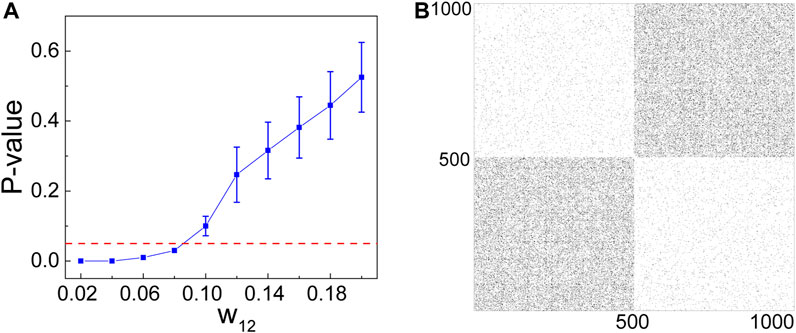FIGURE 1. Simulation results of the hypothesis test algorithm for the balanced two-block DCSBM: p-value as a function of the connecting parameter w12 (A) and the illustration of the adjacency matrix for w12 = 0.02 (B). Dashed line corresponds to the significant level α = 0.05.

We proceed by applying our algorithm to the DCSBM with unbalanced blocks. Specifically, we examine the scenario where the two blocks have different sizes, denoted as n1 and n2, respectively. To investigate the impact of community size, we set w12 = w21 = 0.02 and w11 = w22 = 0.2.

Figure 2A illustrates the behavior of the p-value as n1 increases from 50 to 100. Notably, the p-value consistently decreases with the growth of n1. This trend is straightforward to comprehend as the detection of the planted block becomes increasingly easier with a larger n1. In fact, the DCSBM demonstrates a clear block structure when n1 ≥ 77. In contrast, in Figure 2B, we set n1 = 100 and plot the p-value against the varying values of w12. Here, an interesting observation emerges: the p-value displays a consistent rise with an increase in w12. This outcome aligns with expectations since the graph gradually loses its block structure, particularly noticeable when w12 ≥ 0.068.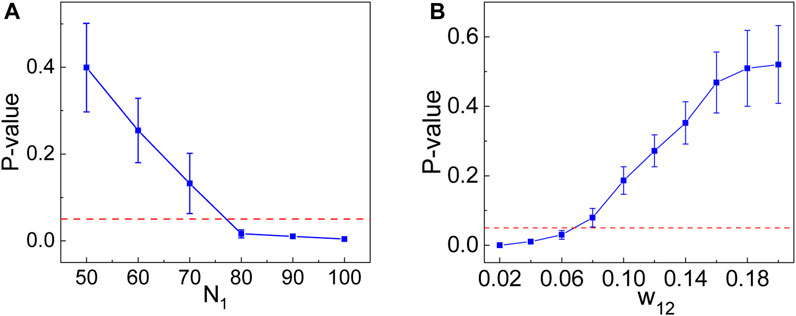FIGURE 2. p-value as a function of n1 (A) and w12 (B) for the unbalanced two-block DCSBM. The dashed lines correspond to the significant level α = 0.05.

## 4 Application to empirical networks

We now apply our algorithm to real-world networks. The first example we consider is the network of a karate club at an American university. This network consists of 34 nodes, and the relationships between these nodes were recorded by Zachary  over a span of 2 years. Due to a disagreement between an instructor (node 0) and an administrator (node 33) regarding class fees, the club ultimately split into two distinct groups. The knowledge of the members within each group makes the karate club network an ideal benchmark for studying community detection.

Upon applying our algorithm to this network, the results obtained are shown in Figure 3A. In the figure, solid circles and squares represent clusters corresponding to instructors and administrators, respectively. Overall, our algorithm successfully splits the vertices in accordance with the known communities, aside from a misclassification of two vertices (nodes 8 and 9) located on the boundary between the two groups. Furthermore, Figure 3B presents a density image of the adjacency matrix, serving as additional confirmation of the block structure within the network.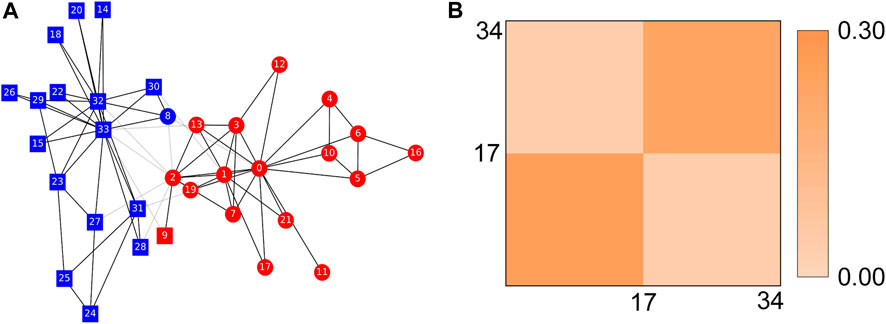FIGURE 3. Performance of the hypothesis test algorithm for the karate club: the illustration of the community division (A) and the density plot for the network (B).

As a second real-world example, we turn our attention to the American college football network . This network is comprised of teams within a league, with each node representing an individual team. Nodes are connected if the corresponding teams played against each other during a specific season. Specifically, our dataset focuses on the 2000 season of the American College Football Division 1-A and includes a total of 115 teams. These teams are organized into 12 conferences, and it is worth noting that games are more commonly played between members of the same conference rather than between teams from different conferences, resulting in a recognizable community structure.

In Figure 4A, we present the community structure obtained through the application of our algorithm to this network. This analysis reveals that the majority of teams have been accurately grouped with other teams from their respective conferences. However, there are a few independent teams that have been assigned to conferences with which they share the closest associations, demonstrating a high level of agreement between the algorithm’s results and the ground truth community structure. Furthermore, Figure 4B displays a density plot of the adjacency matrix, providing further clarity on this phenomenon.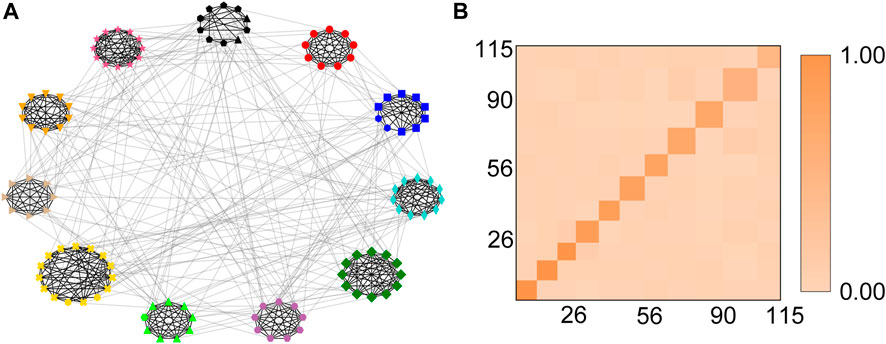FIGURE 4. Community division (A) and density matrix (B) for the American college football network.

To quantitatively compare the results of our algorithm to the ground truth and those of the state-of-the-art methods, we introduce the following two measures: the adjusted Rand index SAR and F1 score. Given two kinds of classifications Pa and Pb, we denote the count of node pairs that classified together in both partitions by q11, classified together in Pa but different in Pb by q10, different in Pa but classified together in Pb by q01, and different in both by q00. It is worth noting that $w11+w10+w01+w00=Cn2=M$, and the adjusted Rand index is defined as follows :

$SAR=w11−1Mw11+w10w11+w0112w11+w10+w11+w01−1Mw11+w10w11+w01.(8)$

Another measure comparing Pa and Pb is F1 score, defined as follows :

$F1=2precisionPa,PbrecallPa,PbprecisionPa,Pb+recallPa,Pb,(9)$

with precision(Pa, Pb) = |PaPb|/|Pb| and recall(Pa, Pb) = |PaPb|/|Pa|.

As depicted in Table 1, our method outperforms state-of-the-art approaches in identifying communities for both real networks. Specifically, we successfully identified two communities in the karate club network and 11 communities in the football network, surpassing the results obtained by other methods. Notably, our approach yielded the highest values for SAR and F1, indicating a superior alignment with the ground truth communities.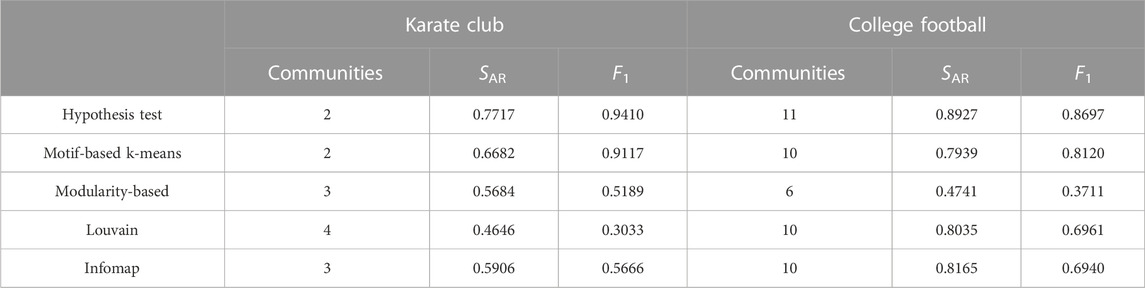TABLE 1. Comparison of the results of the hypothesis test algorithm to the ground truth and those of the state-of-the-art algorithms.

## 5 Conclusion

As a prominent model for the analysis of structural data, the SBM and its variants, particularly the DCSBM, have garnered significant attention in the realm of community detection within networks . The DCSBM, in particular, stands out for its efficacy in handling networks characterized by a highly skewed degree distribution. In this paper, we introduced a novel hypothesis test designed for community detection in complex networks, making dual contributions in terms of both model and algorithm. On the modeling front, we introduced a graph dissimilarity measure that incorporates the vertex distance distribution, clustering coefficient distribution, and alpha-centrality distribution. Utilizing this dissimilarity measure between the DCSBM and DCERG, we proposed a hypothesis testing statistic. In the algorithmic domain, we devised a two-stage algorithm. Initially, we determined whether the original network adhered to the DCERG. If not, we iteratively bipartitioned it until each subgraph conformed to the DCERG. A new criterion for bipartitioning was introduced, integrating edge betweenness and edge clustering coefficient. We applied the algorithm to both synthetic and real networks. Overall, the proposed method marks a significant advancement over existing state-of-the-art approaches. It demonstrates feasibility in detecting communities within networks characterized by broad degree distributions, even when the actual number of communities is unknown.

There are several promising directions for future research in this field. One key area involves exploring alternative approaches to measuring graph dissimilarity, as it remains an open problem. Particularly, for networks with higher-order architecture, it would be beneficial to consider measures that go beyond pairwise interactions to enhance the model’s capacity . Additionally, while the Gaussian distribution is commonly chosen for the kernel density distribution, it may be valuable to explore other distributions, like the widely used Epanechnikov distribution in financial data analysis, to cater to specific interests. In terms of computational complexity, a crucial avenue would involve determining the theoretical distribution for dissimilarity, ultimately contributing to significant reductions in computational overhead. Furthermore, the proposed framework can be extended to incorporate more sophisticated block models, such as exponential , multilevel , and dynamic  models, offering additional benefits and insights.

## Data availability statement

The original contributions presented in the study are included in the article/Supplementary Material; further inquiries can be directed to the corresponding author.

## Author contributions

X-JX and JM conceived and designed the study. CC developed the code and performed the simulations. X-JX and JM interpreted the results and wrote the manuscript. All authors contributed to the article and approved the submitted version.

## Acknowledgments

X-JX and CC acknowledge financial support from NSFC 12071281 and STCSM 22JC1401401. JM acknowledges financial support from the project I3N and FCT/MEC UIDB/50025/2020 and UIDP/50025/2020.

## Conflict of interest

The authors declare that the research was conducted in the absence of any commercial or financial relationships that could be construed as a potential conflict of interest.

## Publisher’s note

All claims expressed in this article are solely those of the authors and do not necessarily represent those of their affiliated organizations, or those of the publisher, the editors, and the reviewers. Any product that may be evaluated in this article, or claim that may be made by its manufacturer, is not guaranteed or endorsed by the publisher.

## References